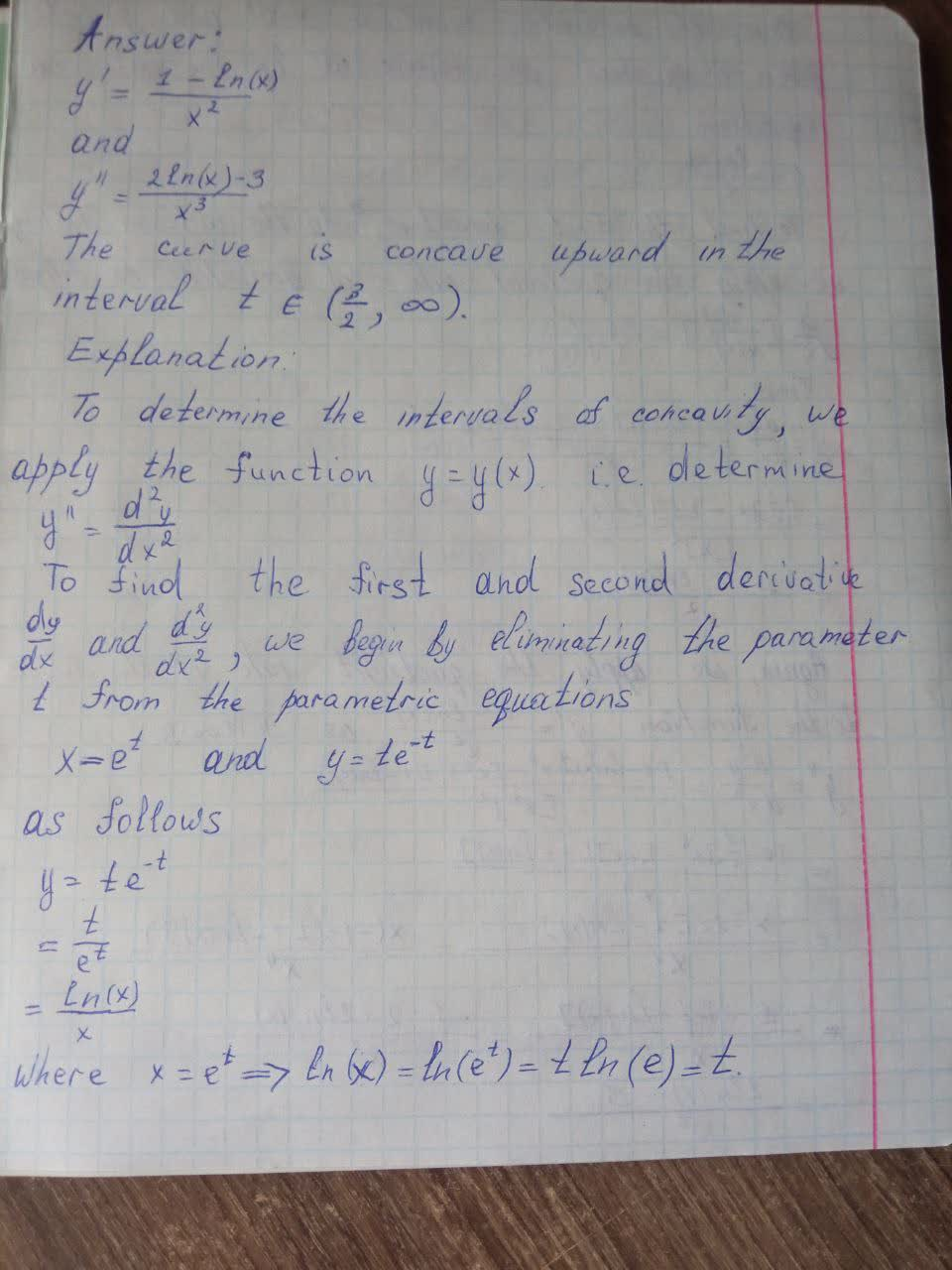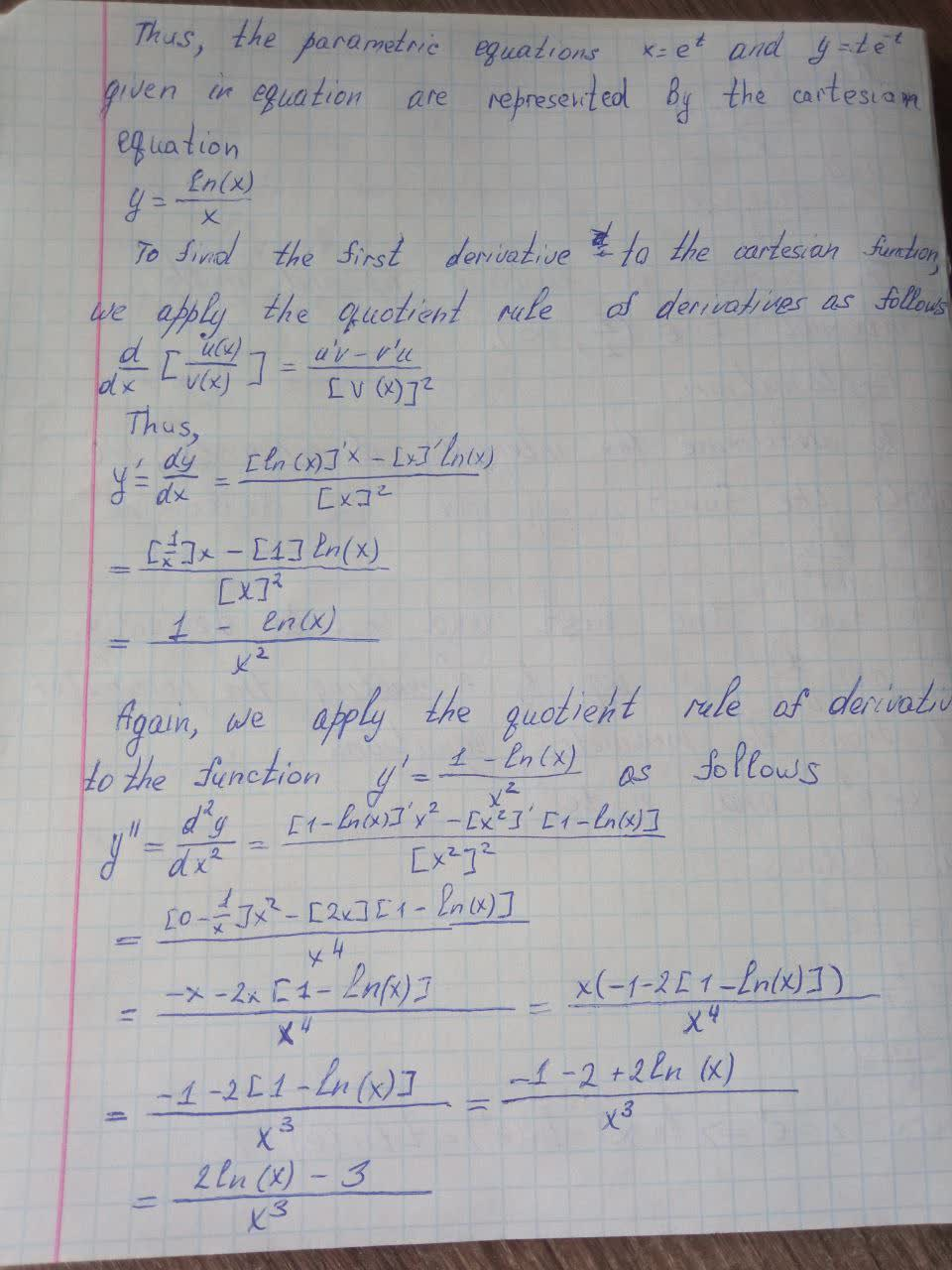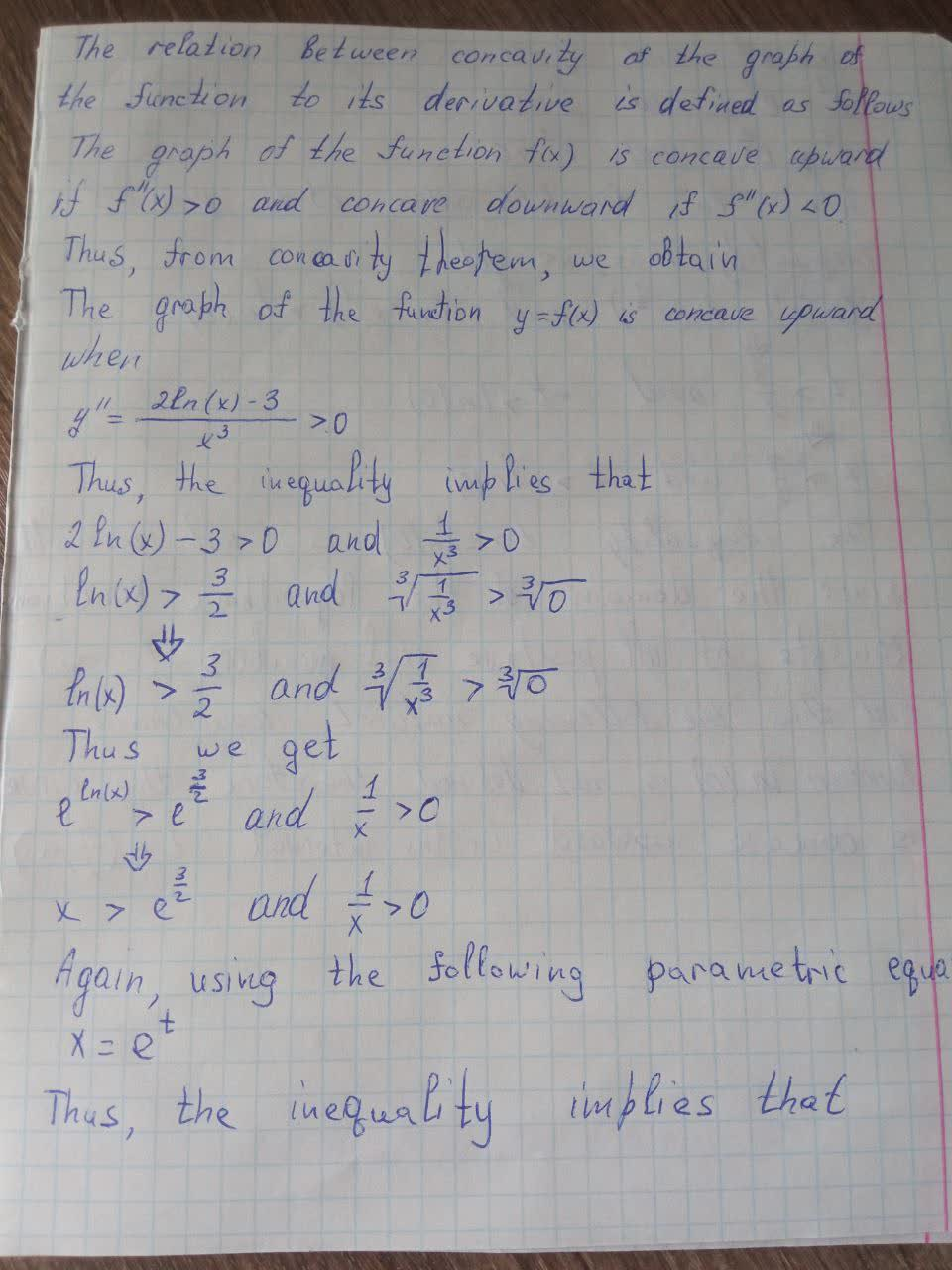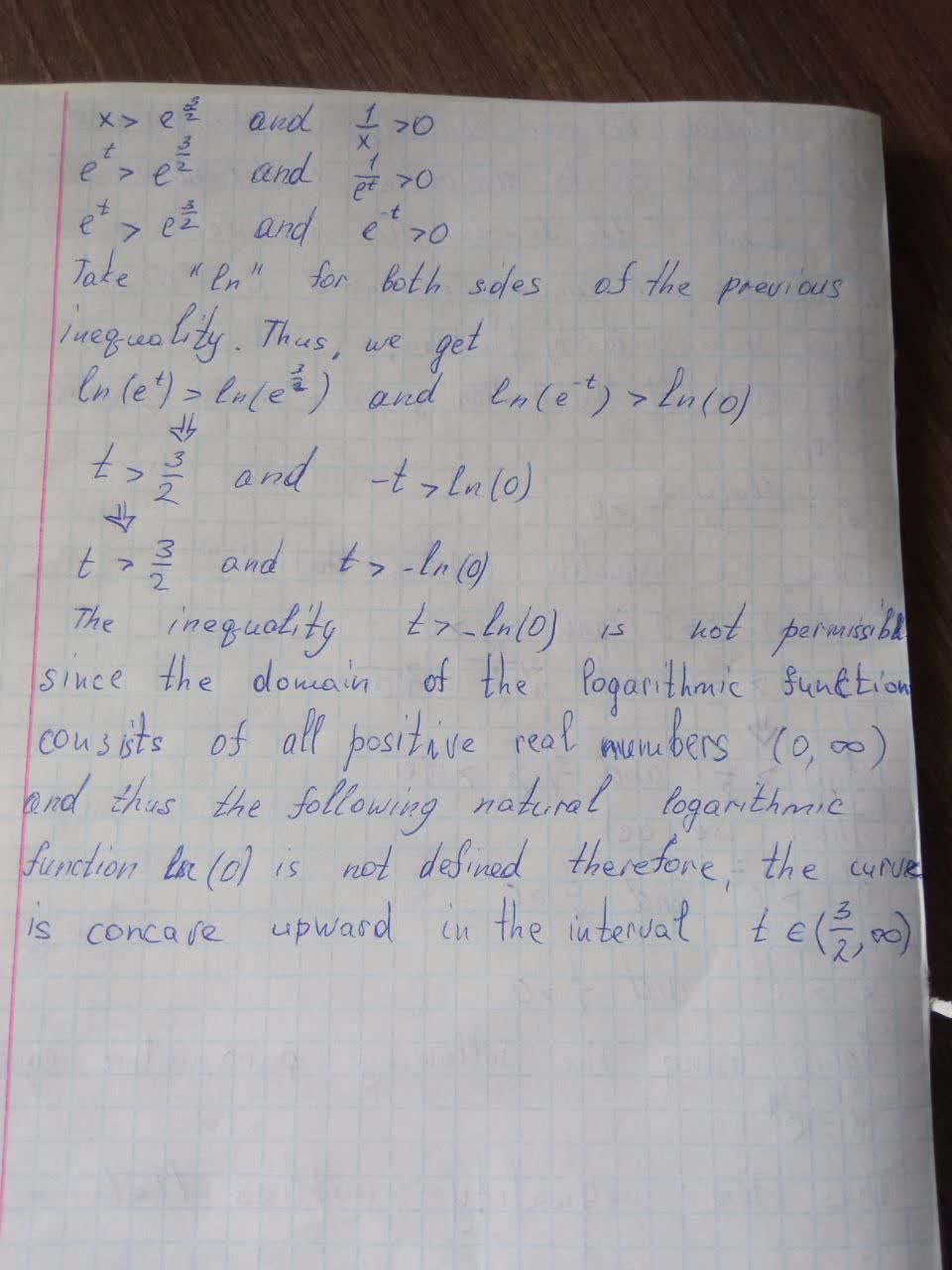# Find \frac{dy}{dx} and \frac{d^2y}{dx^2}.x=e^t,y=te^{-t}. For which values ofmattgondek4 2021-09-11 Answered
Find $$\displaystyle{\frac{{{\left.{d}{y}\right.}}}{{{\left.{d}{x}\right.}}}}$$ and $$\displaystyle{\frac{{{d}^{{2}}{y}}}{{{\left.{d}{x}\right.}^{{2}}}}}.{x}={e}^{{t}},{y}={t}{e}^{{-{t}}}$$. For which values of t is the curve concave upward?

• Questions are typically answered in as fast as 30 minutes

### Plainmath recommends

• Get a detailed answer even on the hardest topics.
• Ask an expert for a step-by-step guidance to learn to do it yourself.AGRFTr
The answer is given below in the photo